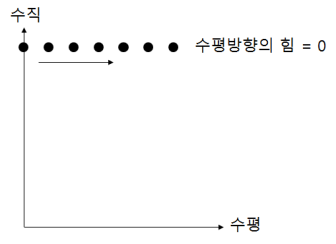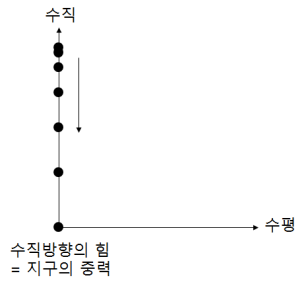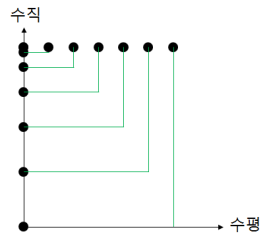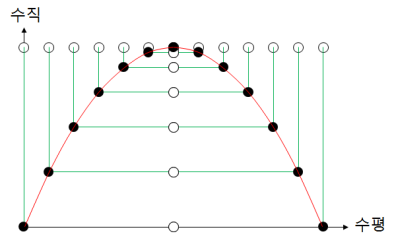# Clay Shooting

## Parabolic motion

Let’s think about the motion of throwing an object horizontally.
There is no horizontal force when there is no air resistance, so there is no change in speed.This time, let’s consider a dropping.
If the initial velocity is ‘0’, the motion that falls vertically is called the free-fall motion. Earth’s gravity is acting in the vertical direction, so a change in speed occurs. In other words, It does ‘accelerated motion.’
Due to the Earth’s gravity, the object accelerates toward the center of the Earth.Assuming that an object is dropped from a moving airplane, it moves linearly at constant velocity in the horizontal direction and free-fall in the vertical direction.
The final motion can be obtained by combining the motion in the horizontal direction and the motion in the vertical direction.What if you throw an object up with an angle?When an object is thrown up with an angle, the only force exerted on the object is gravity, and it moves at a constant velocity in the horizontal direction, resulting in a parabolic orbit.